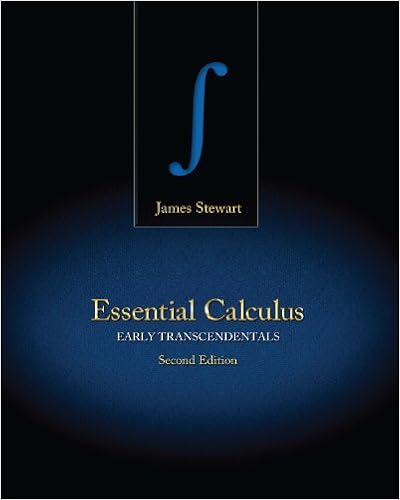# Midterm 2 Practice Solutions - MATH 6BWinter 2016 Vector...

• Test Prep
• 6
• 50% (2) 1 out of 2 people found this document helpful

This preview shows page 1 - 3 out of 6 pages.

##### We have textbook solutions for you!
The document you are viewing contains questions related to this textbook.The document you are viewing contains questions related to this textbook.
Chapter 8 / Exercise 5
Essential Calculus: Early Transcendentals
StewartExpert Verified
MATH 6B–Winter 2016Vector Calculus IIStudy Guide Midterm 2: Extra Problems1. Recognize a geometric series. In the following examples, give a check mark to the geometric series andtwo check marks to the convergent geometric series. Find their sum when possible. If the series is notgeometric, can you determine their convergence/divergence?
2. Identify what is the optimal test to determine convergence of a series. For the following series, write atleast one test that will determine their convergence/divergence, describe how you will use each test, andstate the reason why the test applies.(a)Xn=1n!2nRatio Test
→∞2n+1n!2n= limn→∞(b)Xn=11n3+ 1Limit Comparison Test
##### We have textbook solutions for you!
The document you are viewing contains questions related to this textbook.The document you are viewing contains questions related to this textbook.
Chapter 8 / Exercise 5
Essential Calculus: Early Transcendentals
StewartExpert Verified
(c)Limit ComparisonTestCompare it withbn= 1/n3, thenlimn1→∞rn3
•••